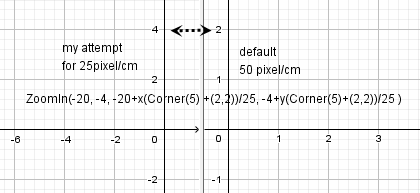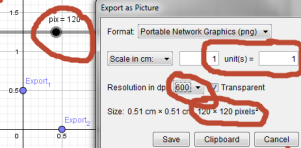# scale x and y axis using pixels

carlosgomes shared this question 8 years ago

Hi all:

Sometimes we have a picture of a graph (created with other software) and want to show that a certain mathematical model fits it. For this, it is necessary that we have a tool that measures the distance between two points in pixels and that Geogebra can scale x and y axis also in pixels.With that implemented (witch I think is very easy), a new way of working with models with kids will be very interesting...1

Hi,

it's easier to scale the image -- if the graph goes from x0 to x1 and from y0 to y1, in its properties change corner 1 to (x0,y0) and corner2 to (x1,y0).1

I have another suggestion to that.

What about being able to "zoom 100%". That would mean that one distance unit in Geogebra also represent a pixel on the screen?1

You can do the "zoom 100%" thing via ZoomOut[x(Corner)/x(Corner-Corner)].1

This seems like a reasonable solution, except there are often small errors of a few pixels. For instance, in the attached file, the distance between each grid line *should* be 400 pixels but *instead* is 401 pixels. Similar issues happen when you use the Export_1 and Export_2 points to set the drawing size, which can be frustrating. When combined, both problems become even more frustrating.

I wish we could set axes and drawing sizes explicitly in the drawing properties. (Is this possible in GSP and CaR?)

In GSP at least you can set the coordinate and measurement units to "pixels".

In CaR you can set the drawing size *and* the coordinate axes size to pixels.1

control the pixel and grid with ZoomIn( <Min x>, <Min y>, <Max x>, <Max y> )1

Not sure how that is helpful. The units are not pixels.1

The problem of difference is (probable):

The dimension of Corner(5) is (2,2) pixel lower then

(Distance(Corner(1), Corner(2)), Distance(Corner(1), Corner(4))) (== the visible windows).

With other words Corner(5) has a rim/gap from 1 pixel around to the visible windows.

-----------------

Length of 1 X-pixel is exact: Distance(Corner(1), Corner(2))/x(Corner(5)+(2,2))

Length of 1 Y-pixel is exact: Distance(Corner(1), Corner(4))/y(Corner(5)+(2,2))

With other words: Corner(5)+(2,2) is the correct value for the visible pixels.1

with Rami info:

suppose you want 75 pixels/cm (grid of 1x1) you can try

ZoomIn(-5, -4, -5+x(Corner(5)+(2,2))/75, -4+y(Corner(5)+(2,2))/75 )1

graphics 1..................................................................graphics2 , same worksheet1

I am not sure I understand 100%. (English translation issue IMO.) One thing I noticed is that when I use the ZoomIn command, I can no longer pan the worksheet left/right/up/down. How do I "unlock" the worksheet so I can move it again? Thanks.1

Which GeoGebra version are you guys using?1

In case I forget in the future, I would like to say that setting the first coordinate to the Export point works well for me.

ZoomIn(x(Export_1), y(Export_1), x(Export_1)+x(Corner(5)+(2,2))/300, y(Export_1)+y(Corner(5)+(2,2))/300 )

But is there a way to use inches as units instead of centimeters? Displays tend to be configured for dpi, at least on Windows.

The above command seems to set the worksheet to 300 pixels per unit, which is exactly what I want. So never mind.

The above works when exporting as PNG, but there seems to be additional strangeness when exporting as SVG. I will start a new question.1

The "strangeness" with respect to SVG output seems to have been resolved in GeoGebra 6. See this thread.1

Quote: "One thing I noticed is that when I use the ZoomIn command, I can no longer pan the worksheet left/right/up/down. How do I "unlock" the worksheet so I can move it again? Thanks."

I would still like an answer for this question, thanks. How does one "unlock" the worksheet after using the ZoomIn command?

What I did to resolve this is create a slider named "Zoom" from 0 to 500. Then I executed this command:

ZoomIn(x(Export_1), y(Export_1), x(Export_1)+x(Corner(5)+(2,2))/Zoom, y(Export_1)+y(Corner(5)+(2,2))/Zoom )

Afterward, I could control the pixel dimensions using the slider. This works well, but I would still like to know how to quickly unlock zooming and panning when needed.1

If you instead use this command:

ZoomIn(CopyFreeObject(x(Export_1)), CopyFreeObject(y(Export_1)), CopyFreeObject(x(Export_1)+x(Corner(5)+(2,2))/Zoom), CopyFreeObject(y(Export_1)+y(Corner(5)+(2,2))/Zoom) )

then the view will never become locked in the first place. See this thread.1

if a=-10 and you use ZoomIn(a, -4, 5,7 ) the zoomin is locked because it depends of a

if you use ZoomIn(-10 , -4, 5,7 ) or ZoomIn(CopyFreeObject(a), -4, 5,7 ) zoomin is unlocked because numbers are free1

Hi kondr:

lets see if you understand the main idea: here is a graph token from another softwareit's easier to scale the image -- if the graph goes from x0 to x1 and from y0 to y1, in its properties change corner 1 to (x0,y0) and corner2 to (x1,y0).

Now I want you to put the coordinate axes scaled to those in the picture (to construct a model that fits it). Please show me how to do it with your idea

https://ggbm.at/5472791

Well, my solution is probably not that elegant, but it works (see attached).

https://ggbm.at/5472811

Hi kondr:

I purpose this solution:

https://ggbm.at/5472831

Hi Kondr:

well, in fact your solution is not that ellegant but that is not the reason for my insistence in my solution. The creation of a tool that measures the distance (of two points) in pixels seems to me very natural for applications of this kind (with the possibility to give x and y axis distance in pixels also, obviously): it don't deform the image and the pupils will understand the notion of scale and why it is used; so, the "solution" proposed by me in the last post seems didatically very interesting.

Sorry but I can't understand the solution proposed by Grobe.1

I didn't say that this feature request will be completely turned down (I wouldn't dare, that's something Mike or Markus must decide), I just wanted to show you that the gain of such feature is not big, because you can achieve the same (or similar) results without such tool.

The activity you describe is certainly interesting and it would be nice if we could find a way how to make the axis mapping easier. But it should be done so that GeoGebra doesn't loose anything from its simplicity (e.g. by generalising existing commands) -- new switches / buttons / tools make it harder to use for beginners.1

after xmas I came back1

When I move the slider from the minimum to the maximum and back again repeatedly, the view gradually zooms out further and further over time.